Скачать презентацию Summary of previous weeks BIL 102 Introduction to

288bc2876ae8ea8078f4cdc2f6effb48.ppt

• Количество слайдов: 48Summary of previous weeks BIL 102 Introduction to Scientific & Engineering Computing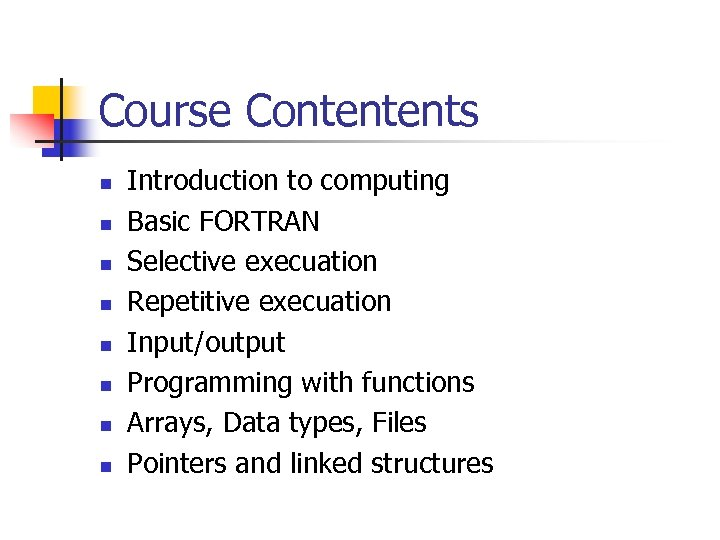Course Contentents n n n n Introduction to computing Basic FORTRAN Selective execuation Repetitive execuation Input/output Programming with functions Arrays, Data types, Files Pointers and linked structures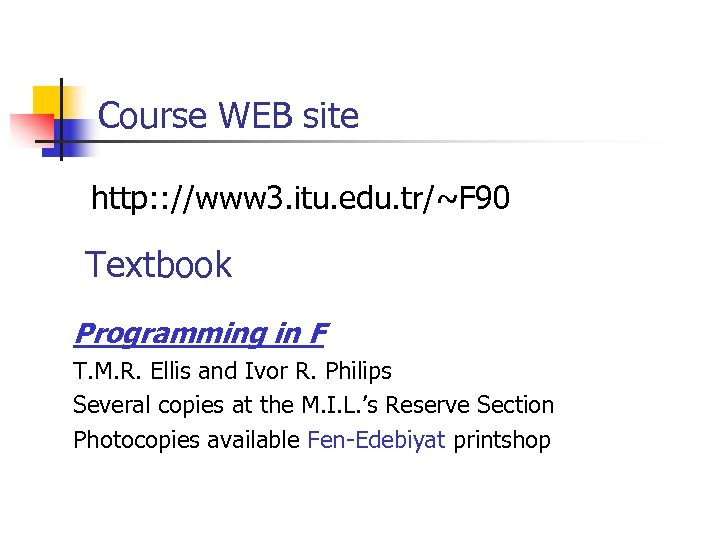Course WEB site http: : //www 3. itu. edu. tr/~F 90 Textbook Programming in F T. M. R. Ellis and Ivor R. Philips Several copies at the M. I. L. ’s Reserve Section Photocopies available Fen-Edebiyat printshop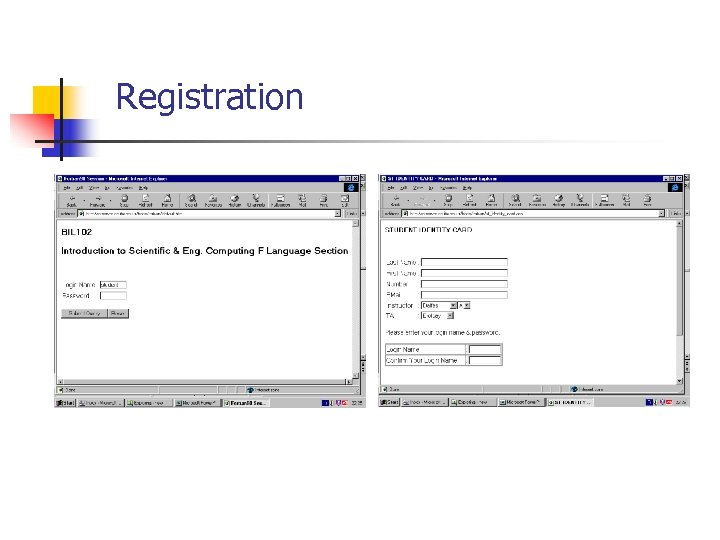Registration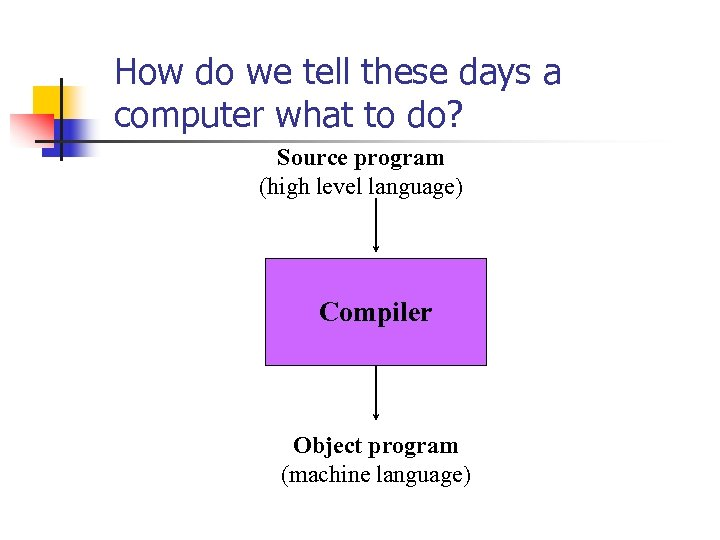How do we tell these days a computer what to do? Source program (high level language) Compiler Object program (machine language)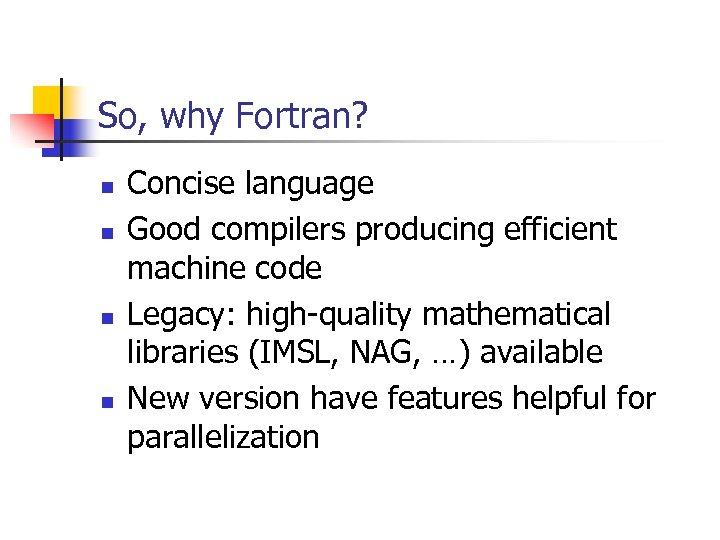So, why Fortran? n n Concise language Good compilers producing efficient machine code Legacy: high-quality mathematical libraries (IMSL, NAG, …) available New version have features helpful for parallelization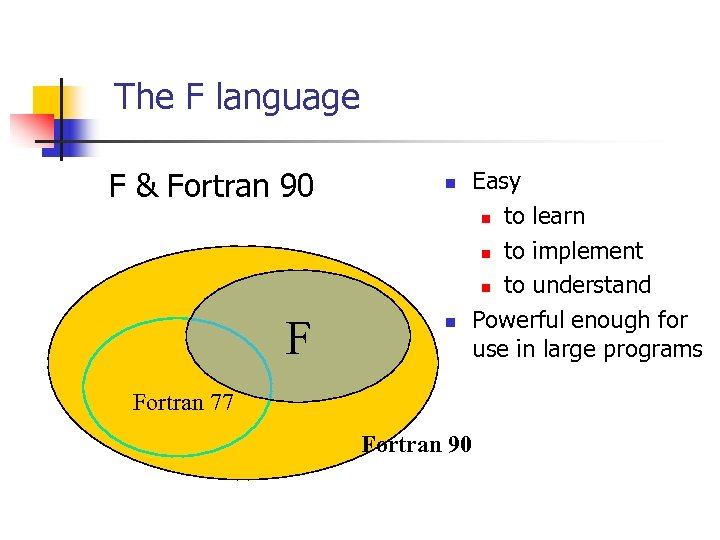The F language F & Fortran 90 F n n Easy n to learn n to implement n to understand Powerful enough for use in large programs Fortran 77 Fortran 90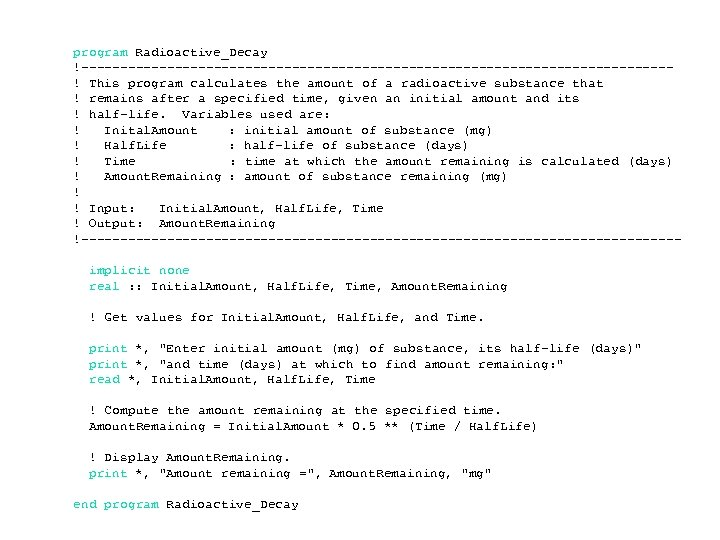program Radioactive_Decay !--------------------------------------! This program calculates the amount of a radioactive substance that ! remains after a specified time, given an initial amount and its ! half-life. Variables used are: ! Inital. Amount : initial amount of substance (mg) ! Half. Life : half-life of substance (days) ! Time : time at which the amount remaining is calculated (days) ! Amount. Remaining : amount of substance remaining (mg) ! ! Input: Initial. Amount, Half. Life, Time ! Output: Amount. Remaining !--------------------------------------implicit none real : : Initial. Amount, Half. Life, Time, Amount. Remaining ! Get values for Initial. Amount, Half. Life, and Time. print *, "Enter initial amount (mg) of substance, its half-life (days)" print *, "and time (days) at which to find amount remaining: " read *, Initial. Amount, Half. Life, Time ! Compute the amount remaining at the specified time. Amount. Remaining = Initial. Amount * 0. 5 ** (Time / Half. Life) ! Display Amount. Remaining. print *, "Amount remaining =", Amount. Remaining, "mg" end program Radioactive_Decay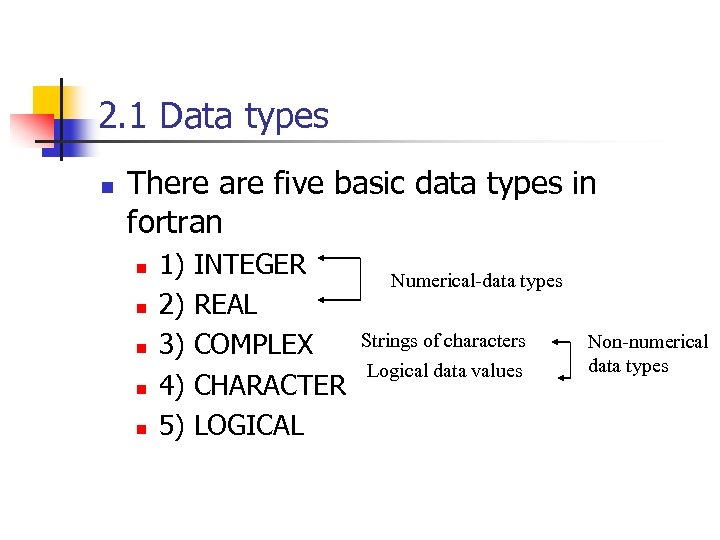2. 1 Data types n There are five basic data types in fortran n n 1) 2) 3) 4) 5) INTEGER REAL COMPLEX CHARACTER LOGICAL Numerical-data types Strings of characters Logical data values Non-numerical data types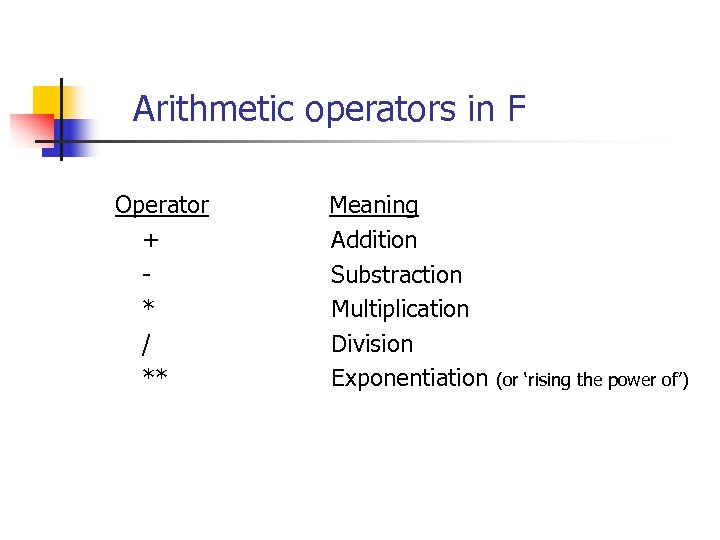Arithmetic operators in F Operator + * / ** Meaning Addition Substraction Multiplication Division Exponentiation (or ‘rising the power of’)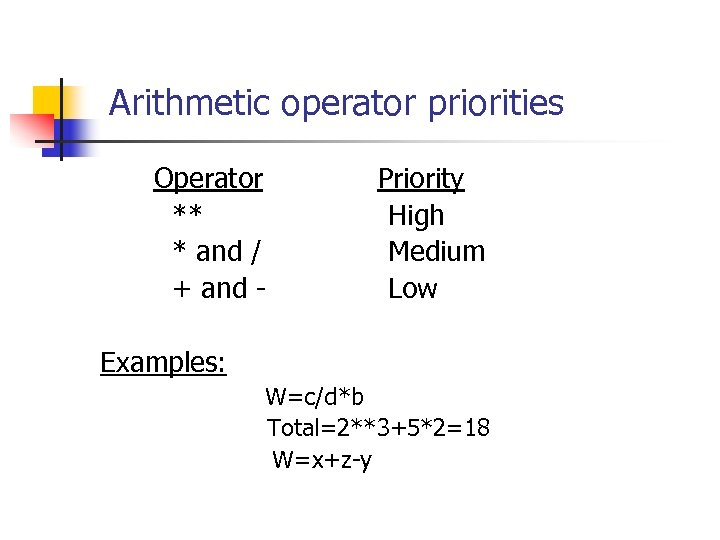Arithmetic operator priorities Operator ** * and / + and - Priority High Medium Low Examples: W=c/d*b Total=2**3+5*2=18 W=x+z-y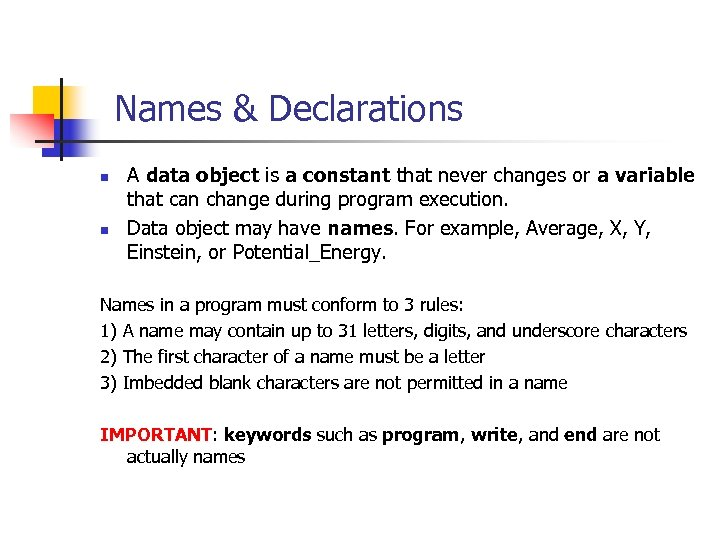Names & Declarations n n A data object is a constant that never changes or a variable that can change during program execution. Data object may have names. For example, Average, X, Y, Einstein, or Potential_Energy. Names in a program must conform to 3 rules: 1) A name may contain up to 31 letters, digits, and underscore characters 2) The first character of a name must be a letter 3) Imbedded blank characters are not permitted in a name IMPORTANT: keywords such as program, write, and end are not actually names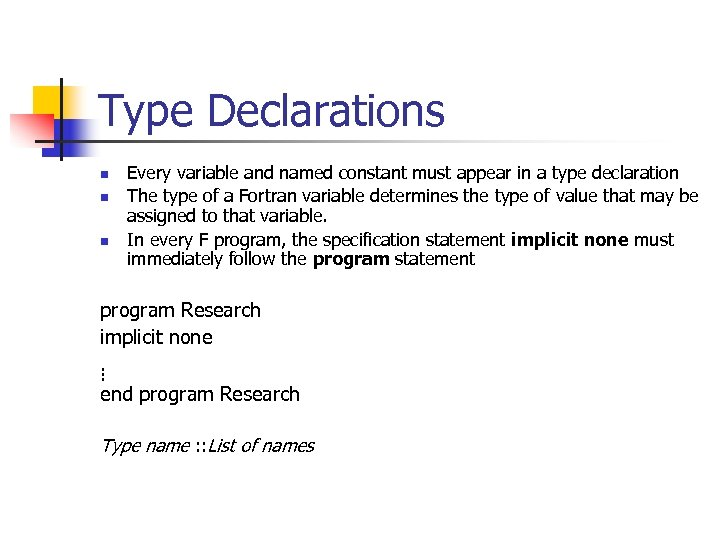Type Declarations n n n Every variable and named constant must appear in a type declaration The type of a Fortran variable determines the type of value that may be assigned to that variable. In every F program, the specification statement implicit none must immediately follow the program statement program Research implicit none. . . end program Research Type name : : List of names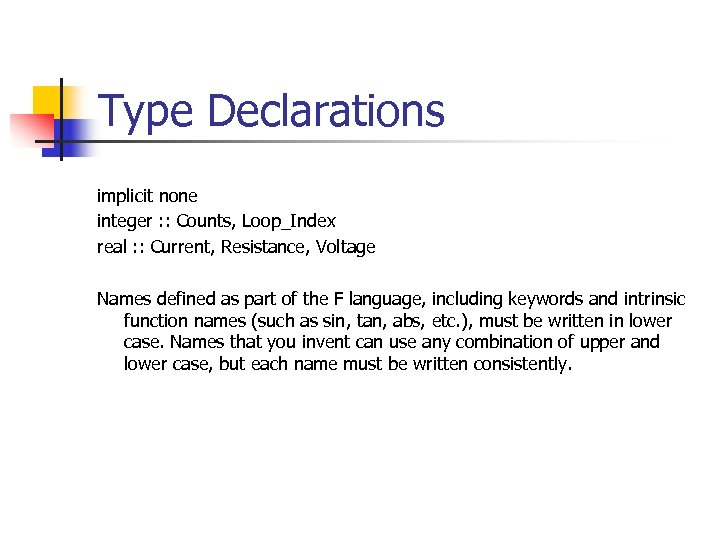Type Declarations implicit none integer : : Counts, Loop_Index real : : Current, Resistance, Voltage Names defined as part of the F language, including keywords and intrinsic function names (such as sin, tan, abs, etc. ), must be written in lower case. Names that you invent can use any combination of upper and lower case, but each name must be written consistently.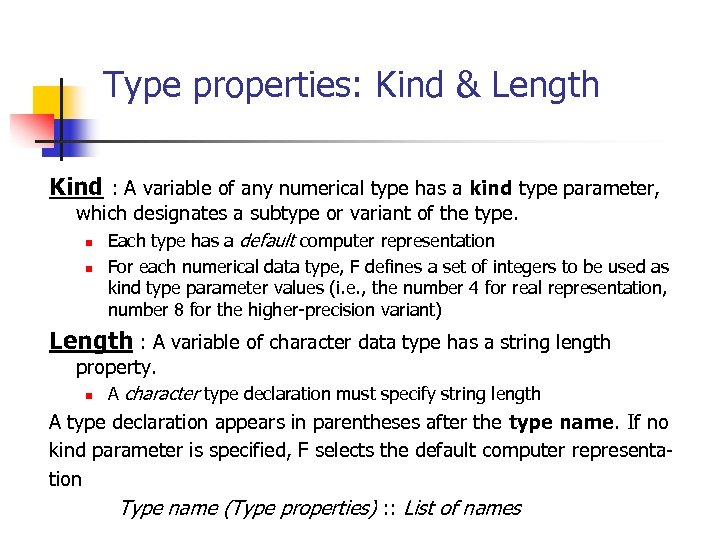Type properties: Kind & Length Kind : A variable of any numerical type has a kind type parameter, which designates a subtype or variant of the type. n Each type has a default computer representation n For each numerical data type, F defines a set of integers to be used as kind type parameter values (i. e. , the number 4 for real representation, number 8 for the higher-precision variant) Length : A variable of character data type has a string length property. n A character type declaration must specify string length A type declaration appears in parentheses after the type name. If no kind parameter is specified, F selects the default computer representation Type name (Type properties) : : List of names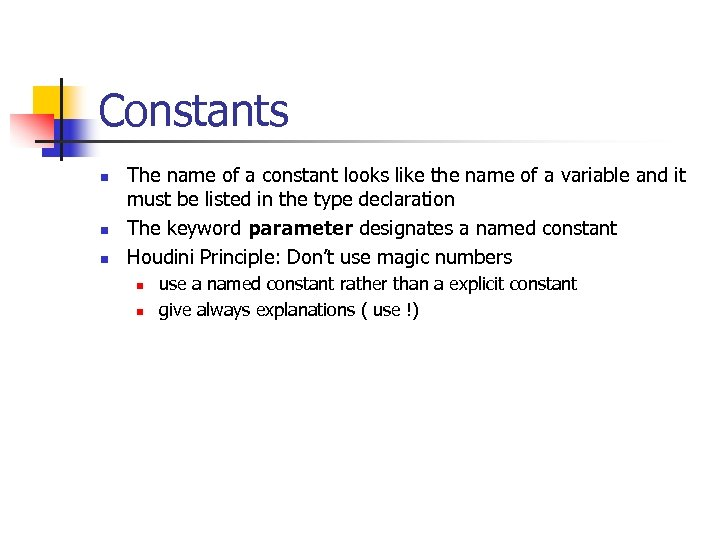Constants n n n The name of a constant looks like the name of a variable and it must be listed in the type declaration The keyword parameter designates a named constant Houdini Principle: Don’t use magic numbers n n use a named constant rather than a explicit constant give always explanations ( use !)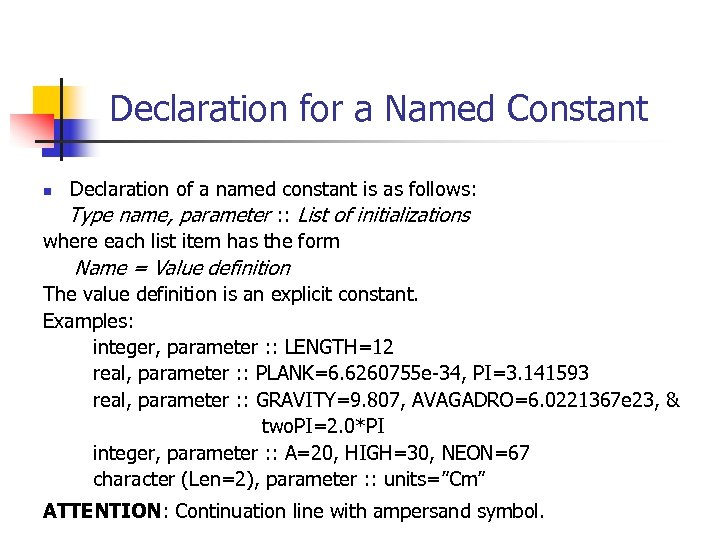Declaration for a Named Constant Declaration of a named constant is as follows: Type name, parameter : : List of initializations where each list item has the form n Name = Value definition The value definition is an explicit constant. Examples: integer, parameter : : LENGTH=12 real, parameter : : PLANK=6. 6260755 e-34, PI=3. 141593 real, parameter : : GRAVITY=9. 807, AVAGADRO=6. 0221367 e 23, & two. PI=2. 0*PI integer, parameter : : A=20, HIGH=30, NEON=67 character (Len=2), parameter : : units=”Cm” ATTENTION: Continuation line with ampersand symbol.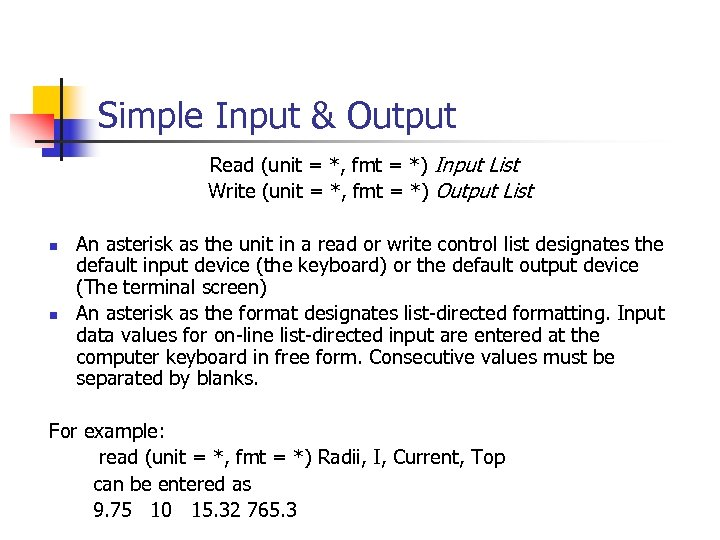Simple Input & Output Read (unit = *, fmt = *) Input List Write (unit = *, fmt = *) Output List n n An asterisk as the unit in a read or write control list designates the default input device (the keyboard) or the default output device (The terminal screen) An asterisk as the format designates list-directed formatting. Input data values for on-line list-directed input are entered at the computer keyboard in free form. Consecutive values must be separated by blanks. For example: read (unit = *, fmt = *) Radii, I, Current, Top can be entered as 9. 75 10 15. 32 765. 3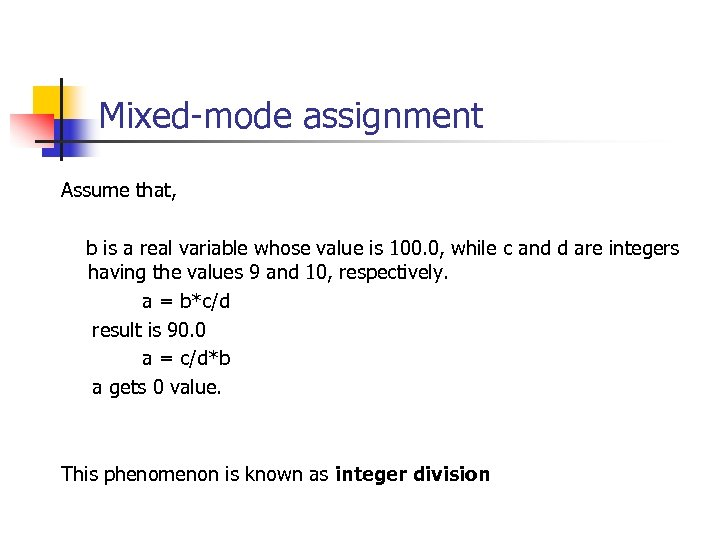Mixed-mode assignment Assume that, b is a real variable whose value is 100. 0, while c and d are integers having the values 9 and 10, respectively. a = b*c/d result is 90. 0 a = c/d*b a gets 0 value. This phenomenon is known as integer division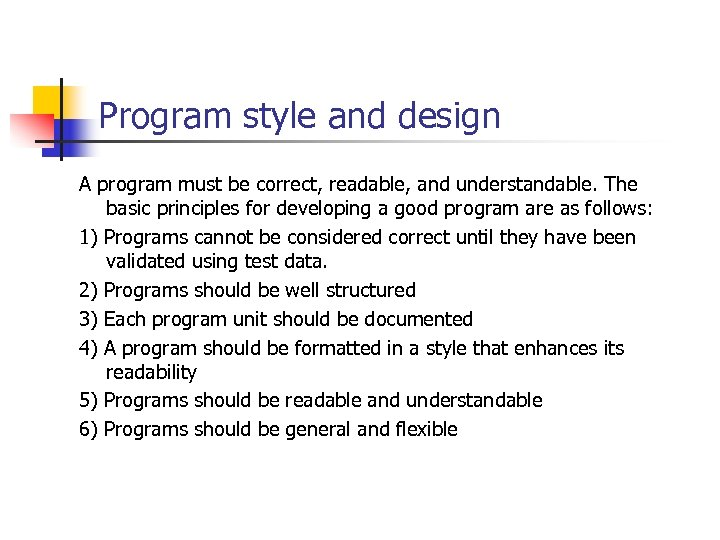Program style and design A program must be correct, readable, and understandable. The basic principles for developing a good program are as follows: 1) Programs cannot be considered correct until they have been validated using test data. 2) Programs should be well structured 3) Each program unit should be documented 4) A program should be formatted in a style that enhances its readability 5) Programs should be readable and understandable 6) Programs should be general and flexible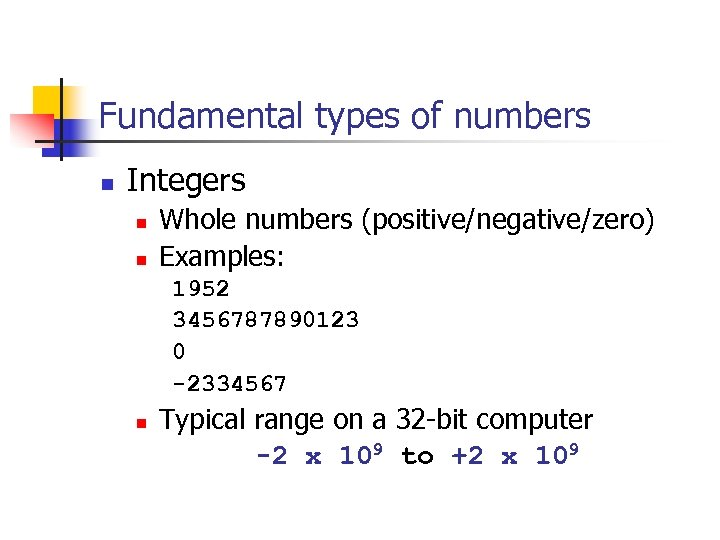Fundamental types of numbers n Integers n n Whole numbers (positive/negative/zero) Examples: 1952 3456787890123 0 -2334567 n Typical range on a 32 -bit computer -2 x 109 to +2 x 109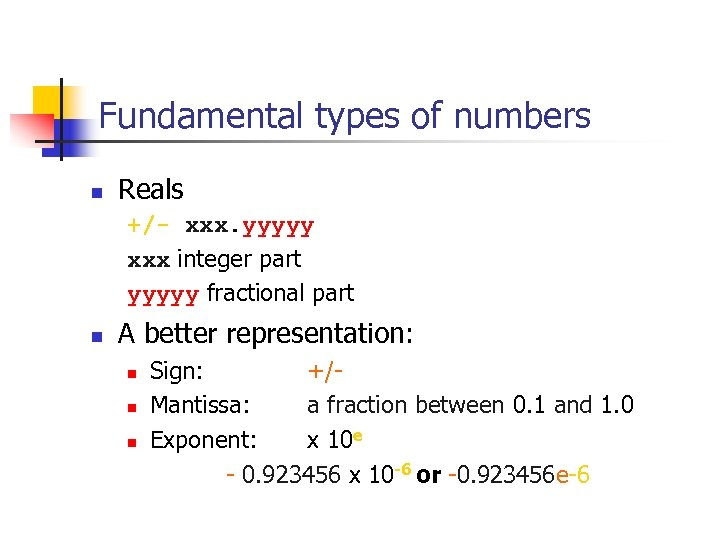Fundamental types of numbers n Reals +/- xxx. yyyyy xxx integer part yyyyy fractional part n A better representation: n n n Sign: +/Mantissa: a fraction between 0. 1 and 1. 0 Exponent: x 10 e - 0. 923456 x 10 -6 or -0. 923456 e-6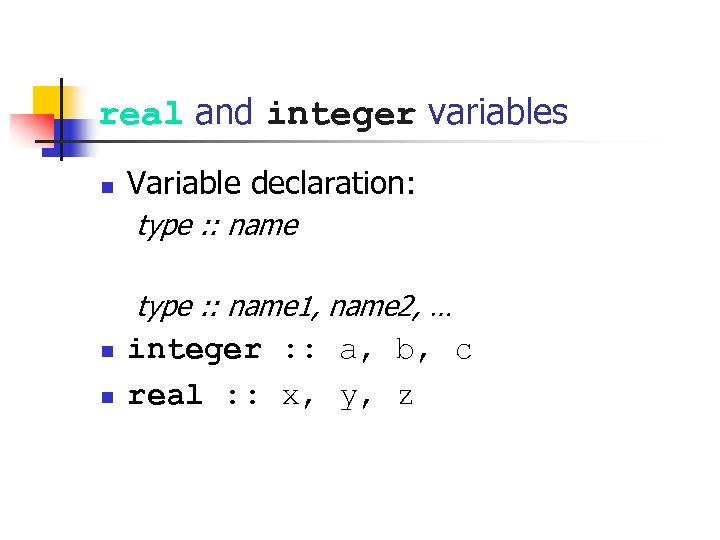real and integer variables n Variable declaration: type : : name 1, name 2, … n n integer : : a, b, c real : : x, y, z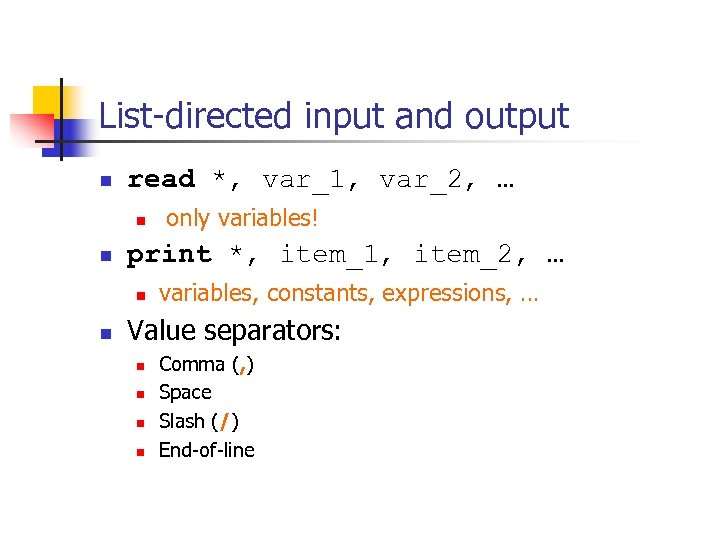List-directed input and output n read *, var_1, var_2, … n n print *, item_1, item_2, … n n only variables! variables, constants, expressions, … Value separators: n n Comma (, ) Space Slash (/) End-of-line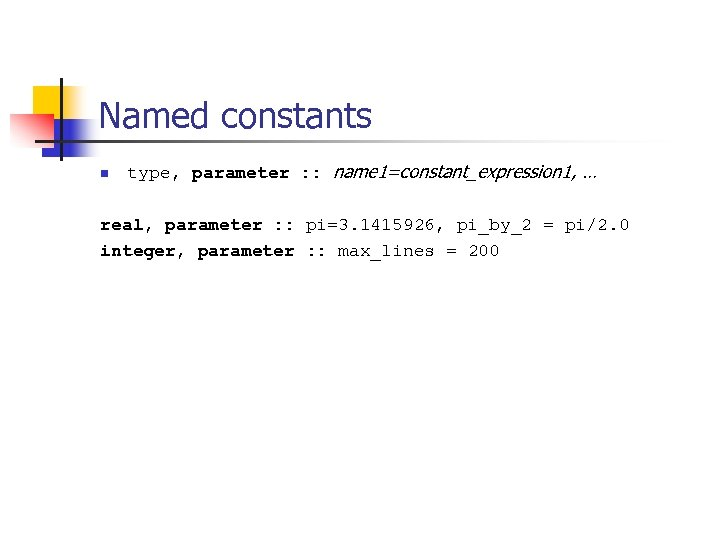Named constants n type, parameter : : name 1=constant_expression 1, … real, parameter : : pi=3. 1415926, pi_by_2 = pi/2. 0 integer, parameter : : max_lines = 200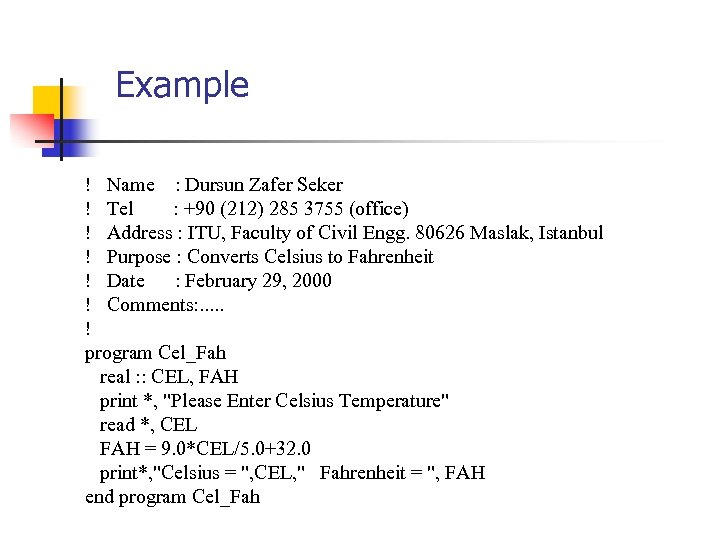Example ! Name : Dursun Zafer Seker ! Tel : +90 (212) 285 3755 (office) ! Address : ITU, Faculty of Civil Engg. 80626 Maslak, Istanbul ! Purpose : Converts Celsius to Fahrenheit ! Date : February 29, 2000 ! Comments: . . . ! program Cel_Fah real : : CEL, FAH print *, "Please Enter Celsius Temperature" read *, CEL FAH = 9. 0*CEL/5. 0+32. 0 print*, "Celsius = ", CEL, " Fahrenheit = ", FAH end program Cel_Fah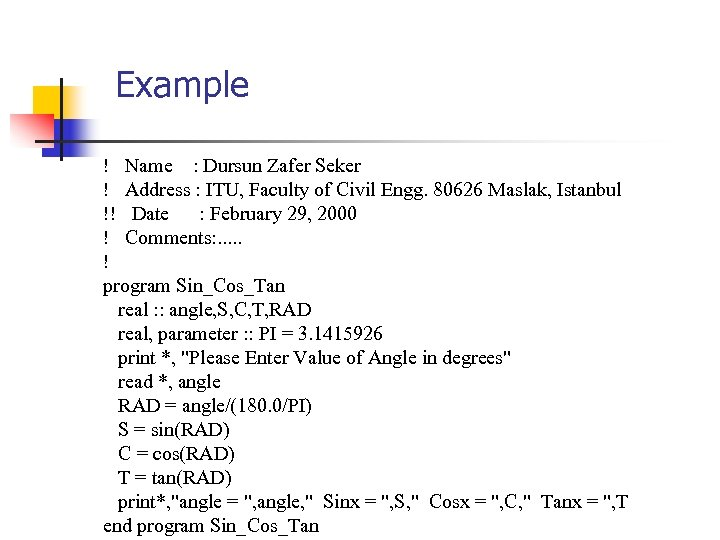Example ! Name : Dursun Zafer Seker ! Address : ITU, Faculty of Civil Engg. 80626 Maslak, Istanbul !! Date : February 29, 2000 ! Comments: . . . ! program Sin_Cos_Tan real : : angle, S, C, T, RAD real, parameter : : PI = 3. 1415926 print *, "Please Enter Value of Angle in degrees" read *, angle RAD = angle/(180. 0/PI) S = sin(RAD) C = cos(RAD) T = tan(RAD) print*, "angle = ", angle, " Sinx = ", S, " Cosx = ", C, " Tanx = ", T end program Sin_Cos_Tan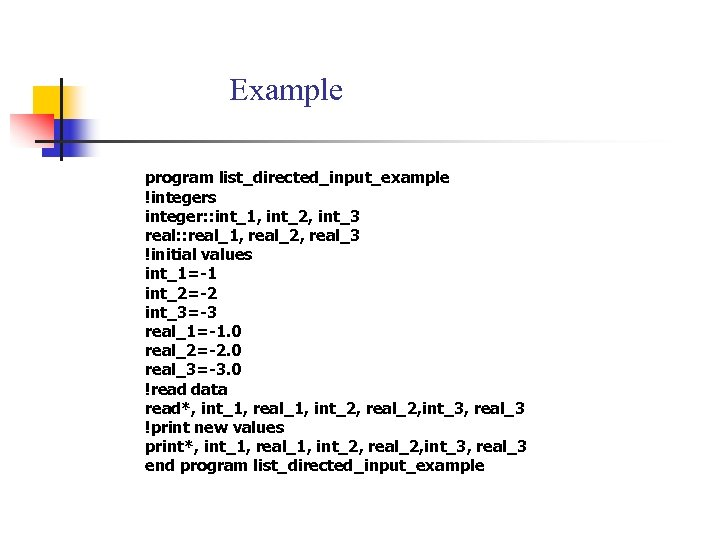Example program list_directed_input_example !integers integer: : int_1, int_2, int_3 real: : real_1, real_2, real_3 !initial values int_1=-1 int_2=-2 int_3=-3 real_1=-1. 0 real_2=-2. 0 real_3=-3. 0 !read data read*, int_1, real_1, int_2, real_2, int_3, real_3 !print new values print*, int_1, real_1, int_2, real_2, int_3, real_3 end program list_directed_input_example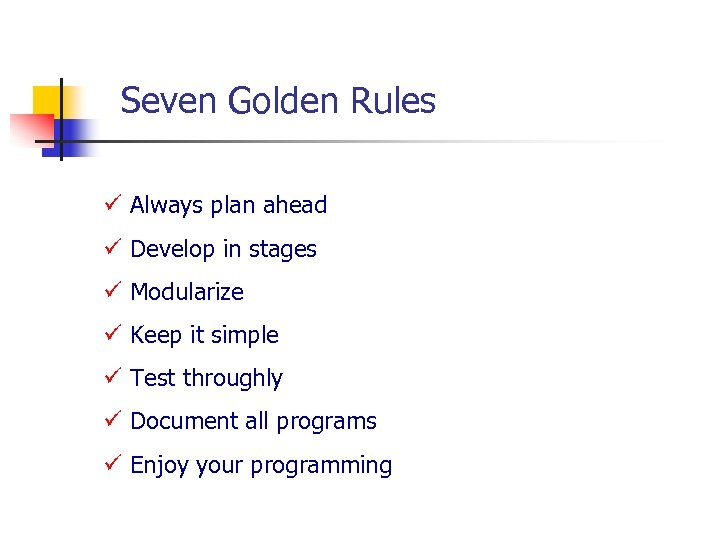Seven Golden Rules ü Always plan ahead ü Develop in stages ü Modularize ü Keep it simple ü Test throughly ü Document all programs ü Enjoy your programming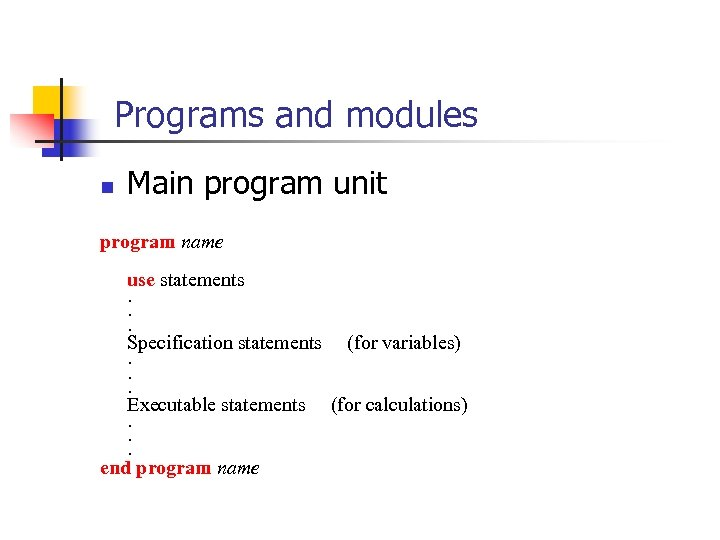Programs and modules n Main program unit program name use statements. . . Specification statements (for variables). . . Executable statements (for calculations). . . end program name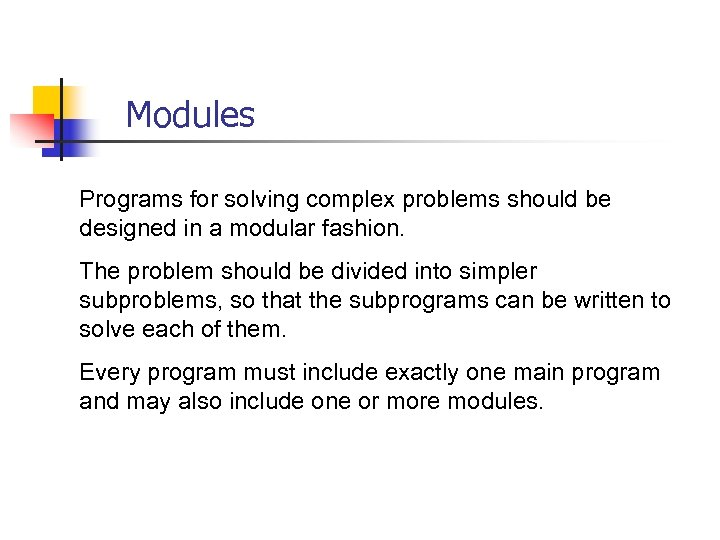Modules Programs for solving complex problems should be designed in a modular fashion. The problem should be divided into simpler subproblems, so that the subprograms can be written to solve each of them. Every program must include exactly one main program and may also include one or more modules.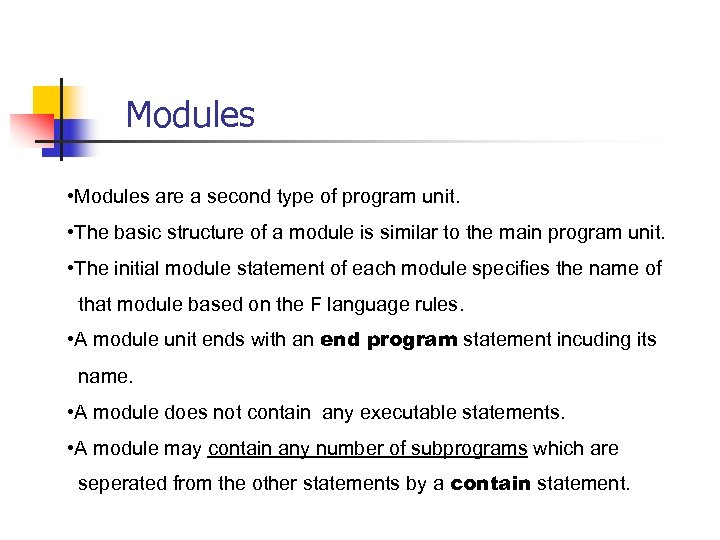Modules • Modules are a second type of program unit. • The basic structure of a module is similar to the main program unit. • The initial module statement of each module specifies the name of that module based on the F language rules. • A module unit ends with an end program statement incuding its name. • A module does not contain any executable statements. • A module may contain any number of subprograms which are seperated from the other statements by a contain statement.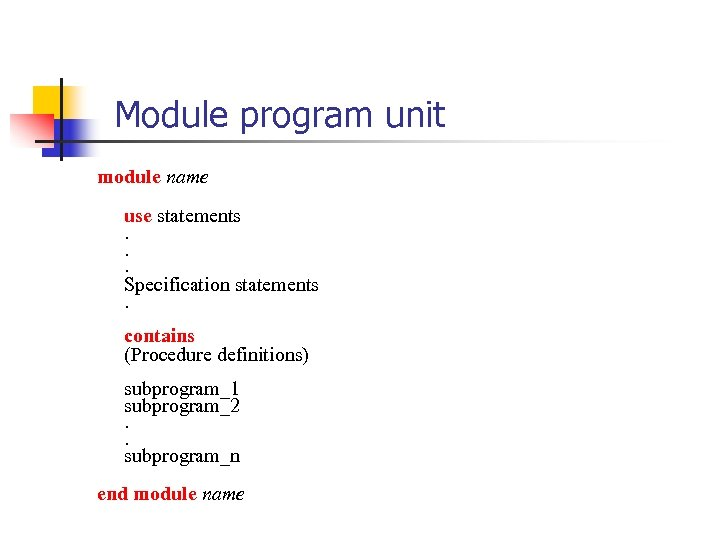Module program unit module name use statements. . . Specification statements. contains (Procedure definitions) subprogram_1 subprogram_2. . subprogram_n end module name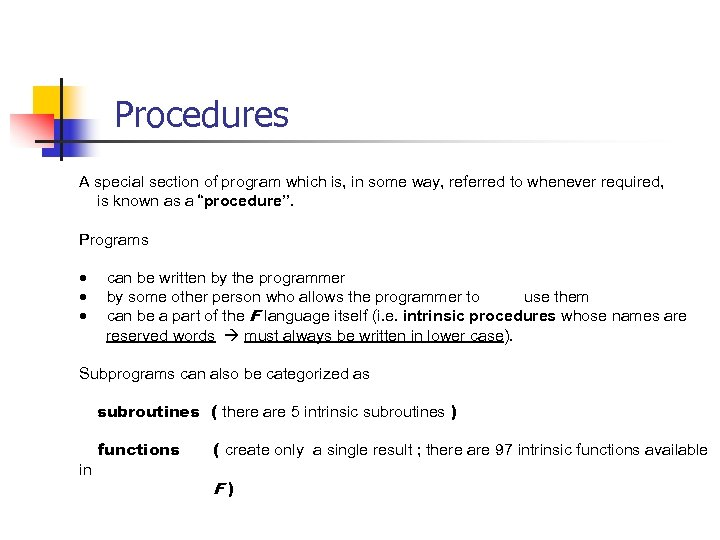Procedures A special section of program which is, in some way, referred to whenever required, is known as a “procedure”. Programs · can be written by the programmer · by some other person who allows the programmer to use them · can be a part of the F language itself (i. e. intrinsic procedures whose names are reserved words must always be written in lower case). Subprograms can also be categorized as subroutines ( there are 5 intrinsic subroutines ) functions ( create only a single result ; there are 97 intrinsic functions available in F )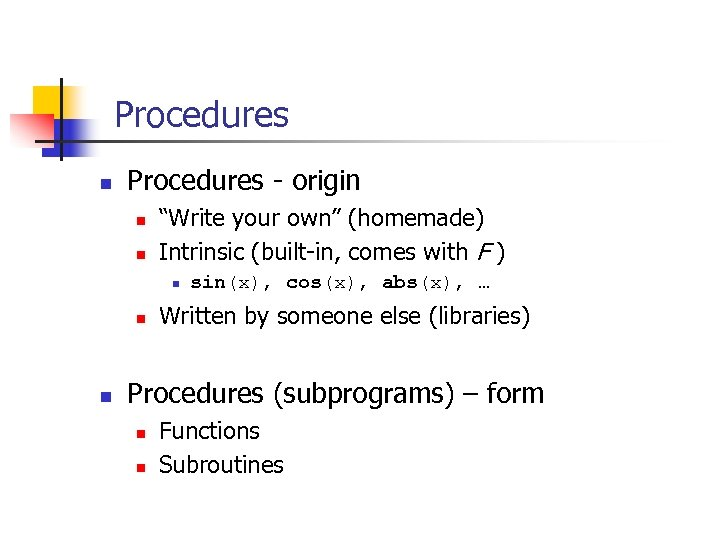Procedures n Procedures - origin n n “Write your own” (homemade) Intrinsic (built-in, comes with F ) n n n sin(x), cos(x), abs(x), … Written by someone else (libraries) Procedures (subprograms) – form n n Functions Subroutines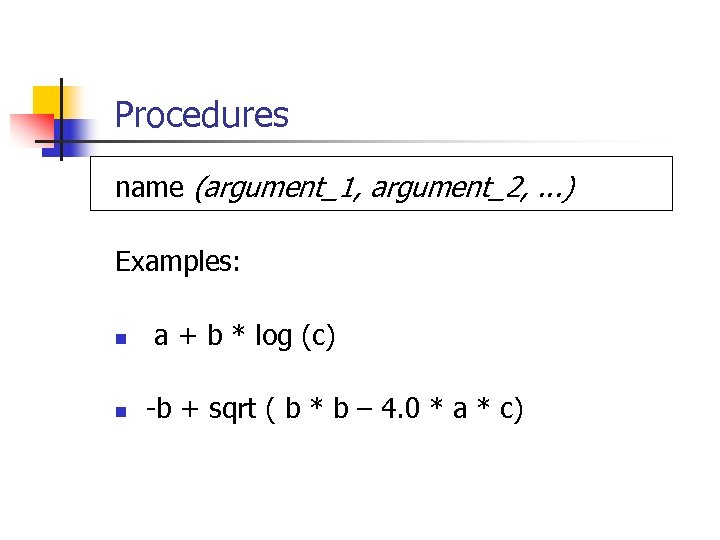Procedures name (argument_1, argument_2, . . . ) Examples: n n a + b * log (c) -b + sqrt ( b * b – 4. 0 * a * c)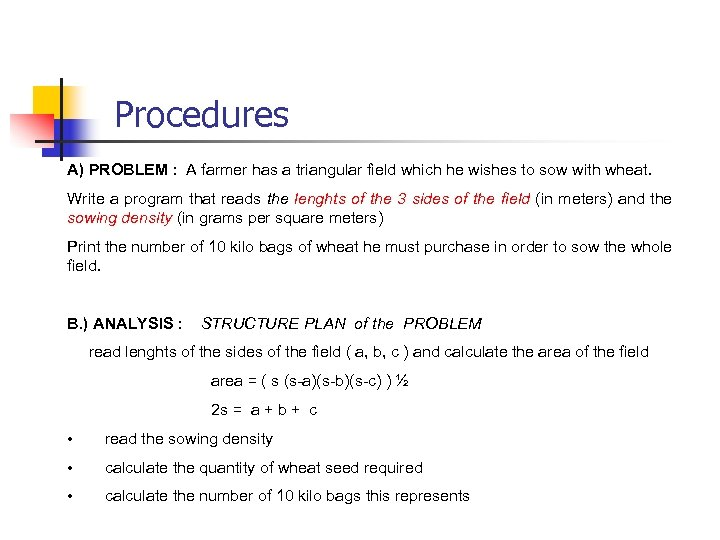Procedures A) PROBLEM : A farmer has a triangular field which he wishes to sow with wheat. Write a program that reads the lenghts of the 3 sides of the field (in meters) and the sowing density (in grams per square meters) Print the number of 10 kilo bags of wheat he must purchase in order to sow the whole field. B. ) ANALYSIS : STRUCTURE PLAN of the PROBLEM read lenghts of the sides of the field ( a, b, c ) and calculate the area of the field area = ( s (s-a)(s-b)(s-c) ) ½ 2 s = a + b + c • read the sowing density • calculate the quantity of wheat seed required • calculate the number of 10 kilo bags this represents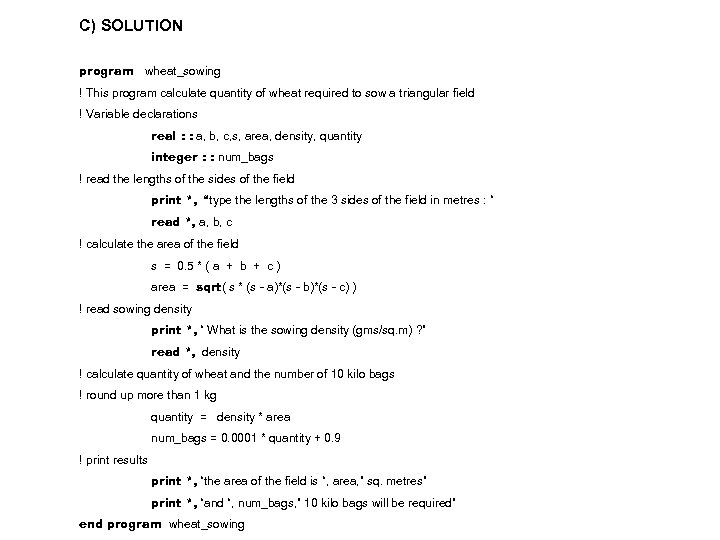C) SOLUTION program wheat_sowing ! This program calculate quantity of wheat required to sow a triangular field ! Variable declarations real : : a, b, c, s, area, density, quantity integer : : num_bags ! read the lengths of the sides of the field print *, “type the lengths of the 3 sides of the field in metres : “ read *, a, b, c ! calculate the area of the field s = 0. 5 * ( a + b + c ) area = sqrt( s * (s - a)*(s - b)*(s - c) ) ! read sowing density print *, “ What is the sowing density (gms/sq. m) ? ” read *, density ! calculate quantity of wheat and the number of 10 kilo bags ! round up more than 1 kg quantity = density * area num_bags = 0. 0001 * quantity + 0. 9 ! print results print *, “the area of the field is “, area, ” sq. metres” print *, “and “, num_bags, ” 10 kilo bags will be required” end program wheat_sowing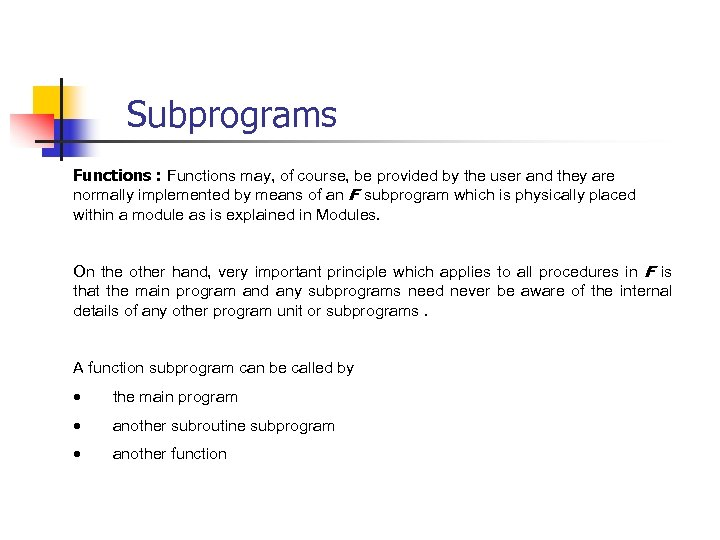Subprograms Functions : Functions may, of course, be provided by the user and they are normally implemented by means of an F subprogram which is physically placed within a module as is explained in Modules. On the other hand, very important principle which applies to all procedures in F is that the main program and any subprograms need never be aware of the internal details of any other program unit or subprograms. A function subprogram can be called by · the main program · another subroutine subprogram · another function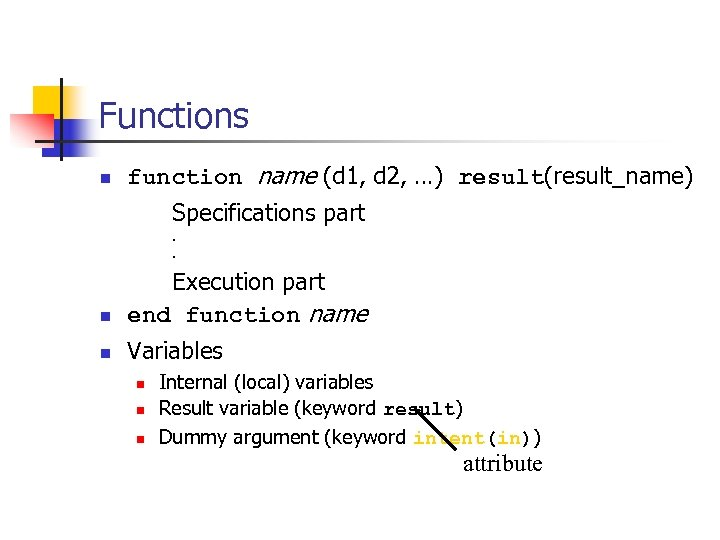Functions n function name (d 1, d 2, …) result(result_name) Specifications part. . n Execution part end function name n Variables n n n Internal (local) variables Result variable (keyword result) Dummy argument (keyword intent(in)) attribute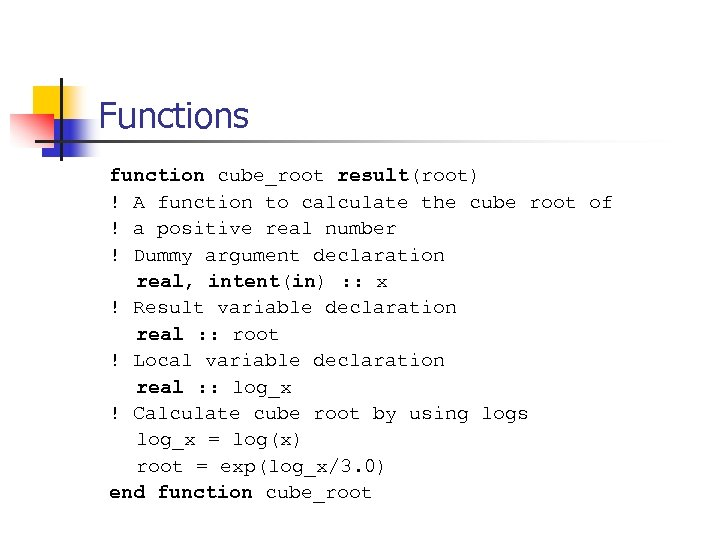Functions function cube_root result(root) ! A function to calculate the cube root of ! a positive real number ! Dummy argument declaration real, intent(in) : : x ! Result variable declaration real : : root ! Local variable declaration real : : log_x ! Calculate cube root by using logs log_x = log(x) root = exp(log_x/3. 0) end function cube_root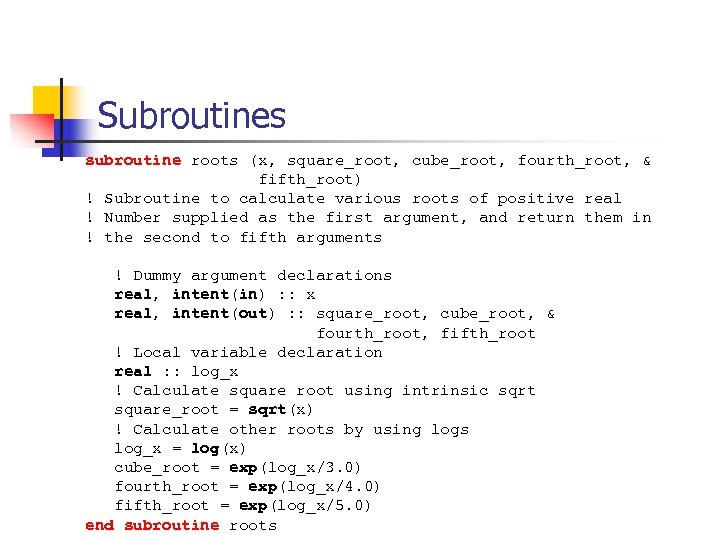Subroutines subroutine roots (x, square_root, cube_root, fourth_root, & fifth_root) ! Subroutine to calculate various roots of positive real ! Number supplied as the first argument, and return them in ! the second to fifth arguments ! Dummy argument declarations real, intent(in) : : x real, intent(out) : : square_root, cube_root, & fourth_root, fifth_root ! Local variable declaration real : : log_x ! Calculate square root using intrinsic sqrt square_root = sqrt(x) ! Calculate other roots by using logs log_x = log(x) cube_root = exp(log_x/3. 0) fourth_root = exp(log_x/4. 0) fifth_root = exp(log_x/5. 0) end subroutine roots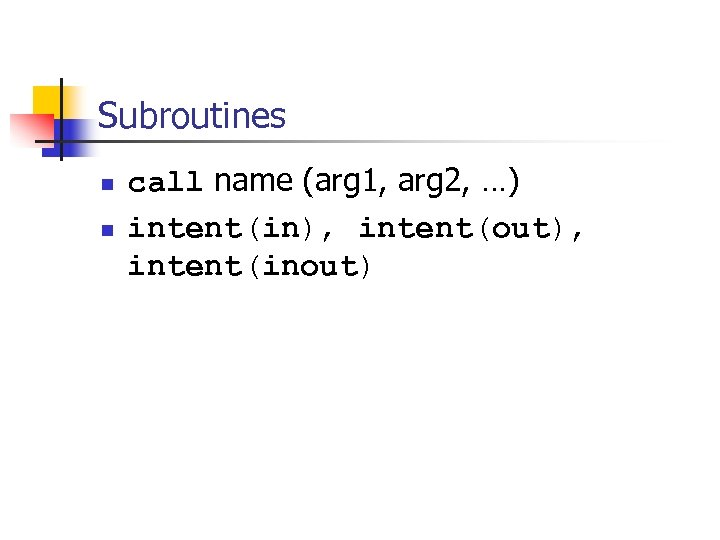Subroutines n n call name (arg 1, arg 2, …) intent(in), intent(out), intent(inout)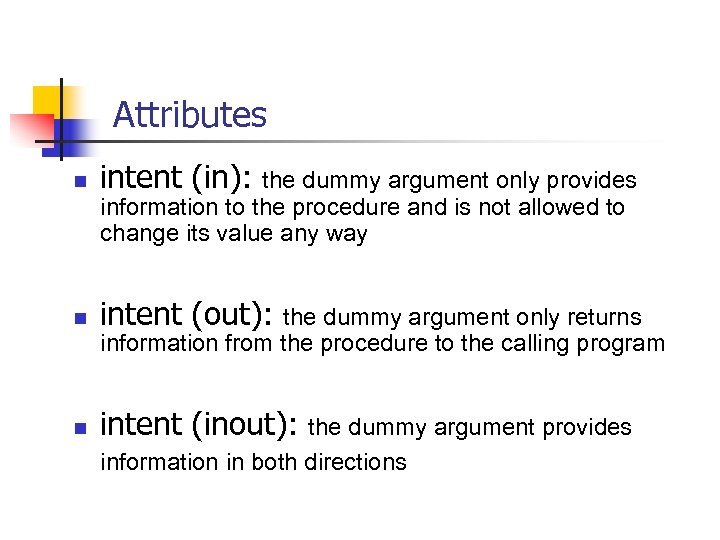Attributes n intent (in): n intent (out): n intent (inout): the dummy argument only provides information to the procedure and is not allowed to change its value any way the dummy argument only returns information from the procedure to the calling program the dummy argument provides information in both directions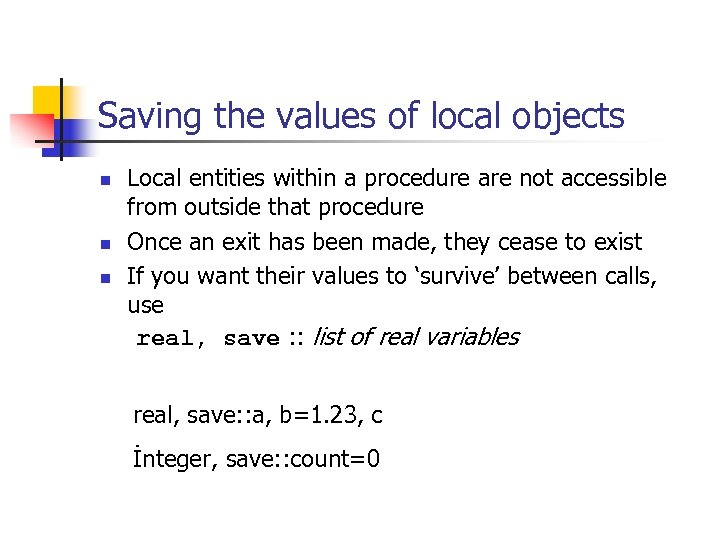Saving the values of local objects n n n Local entities within a procedure are not accessible from outside that procedure Once an exit has been made, they cease to exist If you want their values to ‘survive’ between calls, use real, save : : list of real variables real, save: : a, b=1. 23, c İnteger, save: : count=0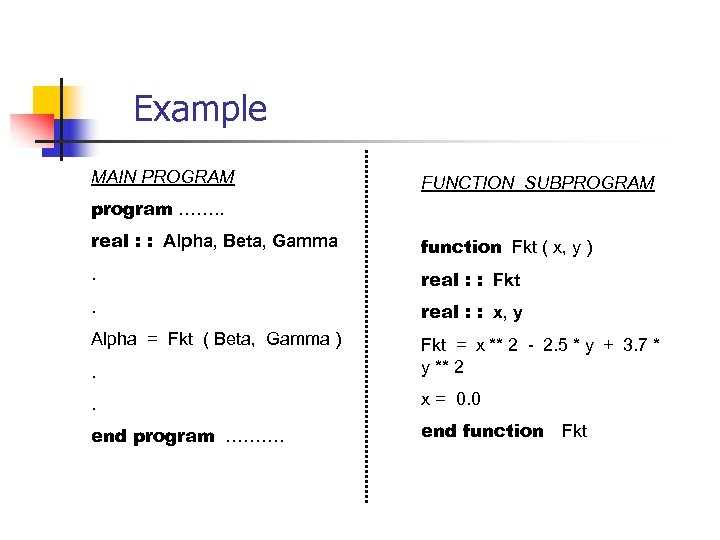Example MAIN PROGRAM FUNCTION SUBPROGRAM program ……. . real : : Alpha, Beta, Gamma function Fkt ( x, y ) . real : : Fkt . real : : x, y Alpha = Fkt ( Beta, Gamma ). Fkt = x ** 2 - 2. 5 * y + 3. 7 * y ** 2 . x = 0. 0 end program ………. end function Fkt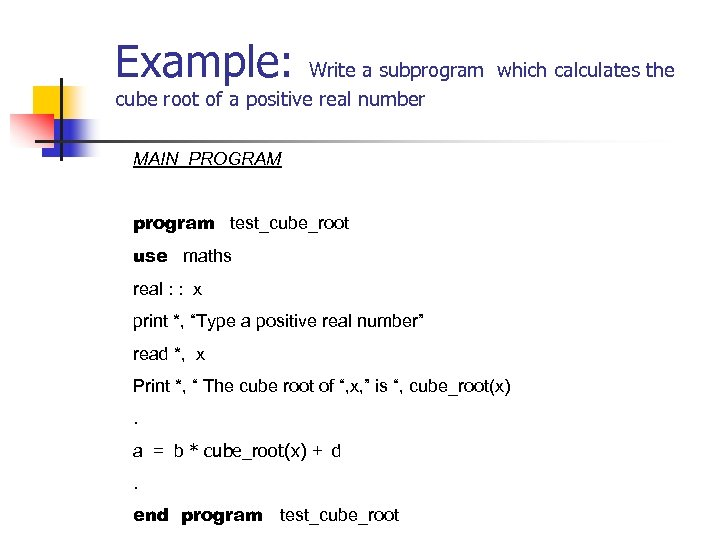Example: Write a subprogram which calculates the cube root of a positive real number MAIN PROGRAM program test_cube_root use maths real : : x print *, “Type a positive real number” read *, x Print *, “ The cube root of “, x, ” is “, cube_root(x). a = b * cube_root(x) + d. end program test_cube_root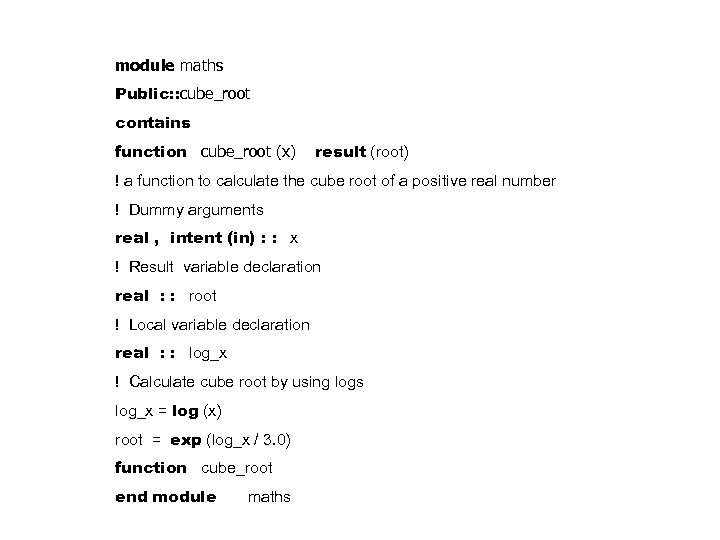module maths Public: : cube_root contains function cube_root (x) result (root) ! a function to calculate the cube root of a positive real number ! Dummy arguments real , intent (in) : : x ! Result variable declaration real : : root ! Local variable declaration real : : log_x ! Calculate cube root by using logs log_x = log (x) root = exp (log_x / 3. 0) function cube_root end module maths